﻿ 树的第五小至第七小度距离 The Fifth to Seventh Minimal Degree Distance of the Tree

Advances in Applied Mathematics
Vol. 08  No. 04 ( 2019 ), Article ID: 29982 , 9 pages
10.12677/AAM.2019.84087

The Fifth to Seventh Minimal Degree Distance of the Tree

Macuo Zhuo, Shuhua Liu, Ji Qiong

Department of Minority Normal School, Qinghai Normal University, Xining Qinghai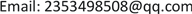Received: Apr. 6th, 2019; accepted: Apr. 21st, 2019; published: Apr. 28th, 2019ABSTRACT

The degree distance indicator of the graph is the sum of the degrees of each point of the graph and the distance between the point and all points of the graph. The tree with the first small to fourth small distance in the order tree is given by He Xiuping, and its degree distance is determined. Based on the careful study of the above results, this paper studies the degree distance sorting problem of the order tree ${T}_{n}$ by using the transformation of the graph, and determines the fifth small to seventh tree in this order, and gives the corresponding degree distance.

Keywords:Graph, Degree Distance, Tree, SortingCopyright © 2019 by authors and Hans Publishers Inc.1. 引言

${\sum }_{u\in V\left(G\right)}\mathrm{deg}\left(u\right)=2m$

${D}^{\prime }\left(G\right)={\sum }_{u\in V\left(G\right)}\mathrm{deg}\left(u\right)D\left(u|G\right)$

$D\left(u|G\right)={\sum }_{v\in V\left(G\right)}d\left(u,v\right)$

2. 预备知识

${D}^{\prime }\left(H\right)>{D}^{\prime }\left(G\right)$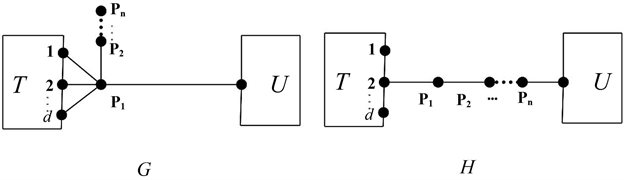Figure 1. Graphs G and H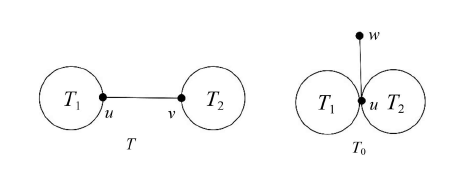Figure 2. Tree T and T0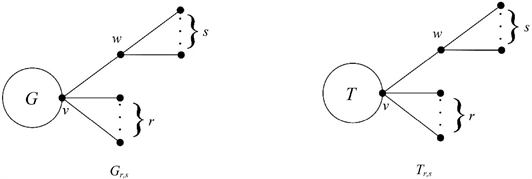Figure 3. Graphs Gr,s and Hr,s

${D}^{\prime }\left({G}_{r,s}\right)>{D}^{\prime }\left({G}_{r+1,s-1}\right)$

(1) 当 $r+m\ge s\ge 1$ 时，有 ${D}^{\prime }\left({T}_{r,s}\right)\ge {D}^{\prime }\left({T}_{r+1,s-1}\right)$

(2) 当 $r+m 时，有 ${D}^{\prime }\left({T}_{r,s}\right)<{D}^{\prime }\left({T}_{r+1,s-1}\right)$

${D}^{\prime }\left(G\right)={\sum }_{u\in V\left(G\right)}\mathrm{deg}\left(u\right)D\left(u|G\right)$

$\begin{array}{c}{D}^{\prime }\left({T}_{r,s}\right)={\sum }_{u\in V\left({T}_{r,s}\right)}\mathrm{deg}\left(u\right)D\left(u|{T}_{r,s}\right)\\ =s\left[2s+3r+2m+D\left(v|T\right)-1\right]+r\left[2r+3s+m+D\left(v|T\right)\right]\\ \text{\hspace{0.17em}}\text{\hspace{0.17em}}+\left(s+1\right)\left[s+2r+m+D\left(v|T\right)\right]+\left[{\mathrm{deg}}_{T}\left(v\right)+\left(r+1\right)\right]\left[r+2s+1+D\left(v|T\right)\right]\\ \text{\hspace{0.17em}}\text{\hspace{0.17em}}+{\sum }_{x\in V\left(T\right)-v}\mathrm{deg}\left(x\right)\left[D\left(v|T\right)+rD\left(x|v\right)+r+sD\left(x|v\right)+2s+D\left(x|v\right)+1\right]\\ =3{\left(s+r\right)}^{2}+4sr+3ms+mr+2\left(s+r+1\right)D\left(x|v\right)+2s+4r+m\\ \text{\hspace{0.17em}}\text{\hspace{0.17em}}+\left(r+2s+1\right){\sum }_{x\in V\left(T\right)}\mathrm{deg}\left(x\right)+{D}^{\prime }\left(T\right)+\left(r+s+1\right){\sum }_{x\in V\left(T\right)-v}D\left(x|v\right)\end{array}$

$\begin{array}{c}{D}^{\prime }\left({T}_{r+1,s-1}\right)=3{\left(s+r\right)}^{2}+4sr+3ms+mr+2\left(s+r+1\right)D\left(x|v\right)+2s+4r+m+{D}^{\prime }\left(T\right)\\ \text{\hspace{0.17em}}\text{\hspace{0.17em}}+\left(r+s+1\right){\sum }_{x\in V\left(T\right)-v}D\left(x|v\right)+\left(r+2s\right){\sum }_{x\in V\left(T\right)}\mathrm{deg}\left(x\right)+4s-4r-2m-2\end{array}$

$\begin{array}{c}{D}^{\prime }\left({T}_{r+1,s-1}\right)-{D}^{\prime }\left({T}_{r,s}\right)=4s-4r-2m-2-{\sum }_{x\in V\left(T\right)}\mathrm{deg}\left(x\right)\\ =4s-4r-2m-2-2\left(m-1\right)\\ =4\left(s-r-m\right)\end{array}$

$r+m\ge s\ge 1$ 时，有 ${D}^{\prime }\left({T}_{r,s}\right)\ge {D}^{\prime }\left({T}_{r+1,s-1}\right)$

$r+m 时，有 ${D}^{\prime }\left({T}_{r,s}\right)<{D}^{\prime }\left({T}_{r+1,s-1}\right)$

${D}^{\prime }\left({T}_{r,s}\right)\ge {D}^{\prime }\left({T}_{r+1,s-1}\right)\ge \cdots \ge {D}^{\prime }\left({T}_{r+s+1,1}\right)$

${D}^{\prime }\left({T}_{r+s-1,1}\right)<{D}^{\prime }\left({T}_{1,r+s-1}\right)<\cdots <{D}^{\prime }\left({T}_{r-1,s+1}\right)<{D}^{\prime }\left({T}_{r,s}\right)$

$m\ge 3$ 时，有

${D}^{\prime }\left({T}_{r+s-1,1}\right)<{D}^{\prime }\left({T}_{r+s-2,2}\right)\le {D}^{\prime }\left({T}_{1,r+s-1}\right)<\cdots <{D}^{\prime }\left({T}_{r-1,s+1}\right)<{D}^{\prime }\left({T}_{r,s}\right)$

$m\ge 4$ 时，有

${D}^{\prime }\left({T}_{r+s-1,1}\right)<{D}^{\prime }\left({T}_{r+s-2,2}\right)<{D}^{\prime }\left({T}_{1,r+s-1}\right)<\cdots <{D}^{\prime }\left({T}_{r-1,s+1}\right)<{D}^{\prime }\left({T}_{r,s}\right)$

$m\ge 2$ 时，只需证 ${D}^{\prime }\left({T}_{r+s-1,1}\right)<{D}^{\prime }\left({T}_{1,r+s-1}\right)$ 成立。

$m\ge 3$ 时， $r+s+m-1>1$$r+s+m-2\ge 2$${D}^{\prime }\left({T}_{r+s-1,1}\right)<{D}^{\prime }\left({T}_{r+s-2,2}\right)$ 成立。

$\begin{array}{c}{D}^{\prime }\left({T}_{r,s}\right)=3{\left(s+r\right)}^{2}+4sr+3ms+mr+2\left(s+r+1\right)D\left(x|v\right)+2s+4r+m\\ \text{\hspace{0.17em}}\text{\hspace{0.17em}}+\left(r+2s+1\right){\sum }_{x\in V\left(T\right)}\mathrm{deg}\left(x\right)+{D}^{\prime }\left(T\right)+\left(r+s+1\right){\sum }_{x\in V\left(T\right)-v}D\left(x|v\right)\end{array}$

$\begin{array}{c}{D}^{\prime }\left({T}_{1,r+s-1}\right)=3{\left(s+r\right)}^{2}+2\left(s+r+1\right)D\left(x|v\right)+{D}^{\prime }\left(T\right)+\left(r+s+1\right){\sum }_{x\in V\left(T\right)-v}D\left(x|v\right)\\ \text{\hspace{0.17em}}\text{\hspace{0.17em}}+6r+6s+3ms+3mr-m-2+2\left(r+s\right){\sum }_{x\in V\left(T\right)}\mathrm{deg}\left(x\right)\end{array}$

$\begin{array}{c}{D}^{\prime }\left({T}_{r+s-2,2}\right)=3{\left(s+r\right)}^{2}+2\left(s+r+1\right)D\left(x|v\right)+{D}^{\prime }\left(T\right)+\left(r+s+1\right){\sum }_{x\in V\left(T\right)-v}D\left(x|v\right)\\ \text{\hspace{0.17em}}\text{\hspace{0.17em}}+12r+12s+ms+mr+5m-20+\left(r+s+3\right){\sum }_{x\in V\left(T\right)}\mathrm{deg}\left(x\right)\end{array}$

${D}^{\prime }\left({T}_{1,r+s-1}\right)-{D}^{\prime }\left({T}_{r+s-2,2}\right)=4\left(m-2\right)\left(r+s-3\right)$

${D}^{\prime }\left({T}_{1,r+s-1}\right)\ge {D}^{\prime }\left({T}_{r+s-2,2}\right)$

$m\ge 3$ 时，有 $4\left(m-2\right)\left(r+s-3\right)>0$，即

${D}^{\prime }\left({T}_{1,r+s-1}\right)>{D}^{\prime }\left({T}_{r+s-2,2}\right)$

${D}^{\prime }\left({T}_{n,1}^{\left(1\right)}\right)<{D}^{\prime }\left({T}_{n,2}^{\left(1\right)}\right)<{D}^{\prime }\left({T}_{n,3}^{\left(1\right)}\right)<\cdots <{D}^{\prime }\left({T}_{n,⌊n/2⌋-1}^{\left(1\right)\right)}$

${D}^{\prime }\left({K}_{1,n-1}\right)<{D}^{\prime }\left({T}_{n,1}^{\left(1\right)}\right)<{D}^{\prime }\left({T}_{n,2}^{\left(1\right)}\right)<{D}^{\prime }\left({T}_{n,1,1}^{\left(2\right)\right)}$

3. 主要结果和证明

$\forall T\in {T}_{n}^{\left(i\right)}$，且 $i>3$，对T做 $i-3$ 次长边变换变成 ${T}^{\prime }$，使 ${T}^{\prime }\in {T}_{n}^{\left(3\right)}$。下面给出 ${T}_{n}^{\left(3\right)}$ 中度距离最小的树，然后对 ${T}_{n}$ 中的树排序。在图4中给出了 ${T}_{n}^{\left(3\right)}$ 中的两类图 ${T}_{k,i,l,j}^{\left(3\right)}$${R}_{k,i,l,j}^{\left(3\right)}$，这些树的度距离的大小与 $k,i,l,m,j$ 的取值有关，这一部分回答了当 $k,i,l,m,j$ 分别取何值时 ${T}_{k,i,l,j}^{\left(3\right)}$${R}_{k,i,l,j}^{\left(3\right)}$ 的度距离取到最小值。

${T}_{k,i,l,j}^{\left(3\right)}\in {T}_{n}^{\left(3\right)}$ 做推论2的变换，得到：

1) 当 $k+l+j+3\ge i$ 时，有 ${D}^{\prime }\left({T}_{k,i,l,j}^{\left(3\right)}\right)\ge {D}^{\prime }\left({T}_{k+1,i-1,l,j}^{\left(3\right)}\right)$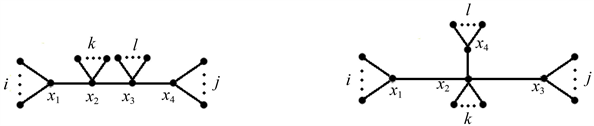Figure 4. Tree ${T}_{k,i,l,j}^{\left(3\right)}$ and ${R}_{k,i,l,j}^{\left(3\right)}$

2) 当 $i>k+l+j+3$ 时，有 ${D}^{\prime }\left({T}_{k,i,l,j}^{\left(3\right)}\right)<{D}^{\prime }\left({T}_{k+1,i-1,l,j}^{\left(3\right)}\right)$

${D}^{\prime }\left({T}_{k,i,l,j}^{\left(3\right)}\right)\ge {D}^{\prime }\left({T}_{k+1,i-1,l,j}^{\left(3\right)}\right)\ge \cdots \ge {D}^{\prime }\left({T}_{k+i-1,1,l,j}^{\left(3\right)\right)}$

${D}^{\prime }\left({T}_{k+i-1,1,l,j}^{\left(3\right)}\right)<{D}^{\prime }\left({T}_{k+i-2,2,l,j}^{\left(3\right)}\right)<{D}^{\prime }\left({T}_{1,k+i-1,l,j}^{\left(3\right)}\right)<{D}^{\prime }\left({T}_{2,k+i-2,l,j}^{\left(3\right)}\right)<\dots <{D}^{\prime }\left({T}_{k,i,l,j}^{\left(3\right)\right)}$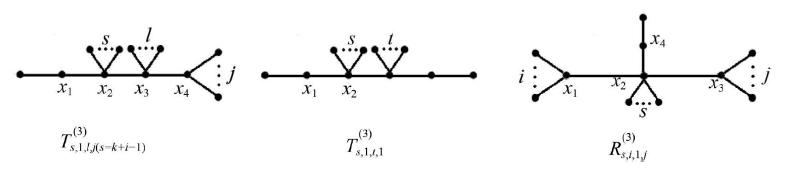Figure 5. Tree ${T}_{s,1,l,j\left(s=k+i-1\right)}^{\left(3\right)}$ ${T}_{s,1,t,1}^{\left(3\right)}$ and ${R}_{s,i,1,j}^{\left(3\right)}$

${D}^{\prime }\left(G\right)={\sum }_{u\in V\left(G\right)}\mathrm{deg}\left(u\right)D\left(u|G\right)$

${D}^{\prime }\left({T}_{s,1,t,1}^{\left(3\right)}\right)=3{\left(s+l+j\right)}^{2}+31\left(s+l+j\right)+4sl+4lj+8sj+4l+16j+60$

$\begin{array}{c}{D}^{\prime }\left({T}_{s,1,l,j}^{\left(3\right)}\right)=3{\left(n-5\right)}^{2}+35\left(n-5\right)-4{\left[s-\left(n-6\right)/2\right]}^{2}-4{\left[j-\left(n-2\right)/2\right]}^{2}\\ \text{\hspace{0.17em}}\text{\hspace{0.17em}}+{\left(n-2\right)}^{2}+{\left(n-6\right)}^{2}+60\end{array}$

${T}_{0,1,n-6,1}^{\left(3\right)},{T}_{1,1,n-7,1}^{\left(3\right)},{T}_{0,1,n-7,2}^{\left(3\right)},{T}_{2,1,n-8,1}^{\left(3\right)},{T}_{1,1,n-8,2}^{\left(3\right)}\left({T}_{0,2,n-7,1}^{\left(3\right)}\right)$

${D}^{\prime }\left({T}_{r,2,l,j}^{\left(3\right)}\right)=3{\left(r+l+j\right)}^{2}+41\left(r+l+j\right)+4l\left(r+j+2\right)+8rj+24j+98$

$l=n-r-j-6$ 代入上式得，

$\begin{array}{c}{D}^{\prime }\left({T}_{r,2,l,j}^{\left(3\right)}\right)=3{\left(n-6\right)}^{2}+41\left(n-6\right)-4{\left[r-\left(n-8\right)/2\right]}^{2}-4{\left[r-\left(n-2\right)/2\right]}^{2}\\ \text{\hspace{0.17em}}\text{\hspace{0.17em}}+{\left(n-8\right)}^{2}+{\left(n-2\right)}^{2}+8n+50\\ =3{n}^{2}+13n-88-4{\left[r-\left(n-8\right)/2\right]}^{2}-4{\left[r-\left(n-2\right)/2\right]}^{2}\\ \text{\hspace{0.17em}}\text{\hspace{0.17em}}+{\left(n-8\right)}^{2}+{\left(n-2\right)}^{2}\end{array}$

$\begin{array}{c}{D}^{\prime }\left({T}_{s,1,l,j}^{\left(3\right)}\right)=3{\left(n-5\right)}^{2}+35\left(n-5\right)-4{\left[s-\left(n-6\right)/2\right]}^{2}-4{\left[j-\left(n-2\right)/2\right]}^{2}\\ \text{\hspace{0.17em}}\text{\hspace{0.17em}}+{\left(n-2\right)}^{2}+{\left(n-6\right)}^{2}+60\end{array}$

${D}^{\prime }\left({T}_{2,1,n-9,2}^{\left(3\right)}\right)=3{n}^{2}+21n-136$${D}^{\prime }\left({T}_{1,1,n-7,1}^{\left(3\right)}\right)=3{n}^{2}+13n-80$${D}^{\prime }\left({T}_{0,1,n-7,2}^{\left(3\right)}\right)=3{n}^{2}+13n-72$

${D}^{\prime }\left({T}_{2,1,n-8,1}^{\left(3\right)}\right)=3{n}^{2}+17n-116$${D}^{\prime }\left({T}_{1,1,n-8,2}^{\left(3\right)}\right)=3{n}^{2}+17n-100$

${D}^{\prime }\left({T}_{0,1,n-6,1}^{\left(3\right)}\right)<{D}^{\prime }\left({T}_{1,1,n-7,1}^{\left(3\right)}\right)<{D}^{\prime }\left({T}_{0,1,n-7,2}^{\left(3\right)}\right)<{D}^{\prime }\left({T}_{2,1,n-8,1}^{\left(3\right)}\right)<{D}^{\prime }\left({T}_{1,1,n-8,2}^{\left(3\right)}\right)<{D}^{\prime }\left({T}_{2,1,n-9,2}^{\left(3\right)\right)}$

${T}_{0,1,n-6,1}^{\left(3\right)},{T}_{1,1,n-7,1}^{\left(3\right)},{T}_{0,1,n-7,2}^{\left(3\right)},{T}_{2,1,n-8,1}^{\left(3\right)},{T}_{1,1,n-8,2}^{\left(3\right)}$

1) 当 $k+i+j+3\ge l$ 时，有 ${D}^{\prime }\left({R}_{k,i,l,j}^{\left(3\right)}\right)\ge {D}^{\prime }\left({R}_{k+1,i,l-1,j}^{\left(3\right)}\right)$

2) 当 $l>k+i+j+3$ 时，有 ${D}^{\prime }\left({R}_{k,i,l,j}^{\left(3\right)}\right)<{D}^{\prime }\left({R}_{k+1,i,l-1,j}^{\left(3\right)}\right)$

${D}^{\prime }\left({R}_{k,i,l,j}^{\left(3\right)}\right)\ge {D}^{\prime }\left({R}_{k+1,i,l-1,j}^{\left(3\right)}\right)\ge \cdots \ge {D}^{\prime }\left({R}_{k+i-1,i,1,j}^{\left(3\right)\right)}$

${D}^{\prime }\left({R}_{k+i-1,i,1,j}^{\left(3\right)}\right)<{D}^{\prime }\left({R}_{k+i-2,i,2,j}^{\left(3\right)}\right)<{D}^{\prime }\left({R}_{1,i,k+i-1,j}^{\left(3\right)}\right)<{D}^{\prime }\left({R}_{2,i,k+i-2,j}^{\left(3\right)}\right)<\cdots <{D}^{\prime }\left({R}_{k,i,l,j}^{\left(3\right)\right)}$

${D}^{\prime }\left({R}_{n-7,1,1,1}^{\left(3\right)}\right)=3{n}^{2}+5n-32$${D}^{\prime }\left({R}_{n-8,2,1,1}^{\left(3\right)}\right)=3{n}^{2}+9n-52$${D}^{\prime }\left({R}_{n-9,2,1,2}^{\left(3\right)}\right)=3{n}^{2}+13n-72$

${D}^{\prime }\left(G\right)={\sum }_{u\in V\left(G\right)}\mathrm{deg}\left(u\right)D\left(u|G\right)$

${D}^{\prime }\left({R}_{s,i,1,j}^{\left(3\right)}\right)=3{\left(i+j+s\right)}^{2}+27\left(i+j+s\right)+4is+8ij+4sj+12i+12j+52$

$s=n-5-i-j$ 代入得

${D}^{\prime }\left({R}_{s,i,1,j}^{\left(3\right)}\right)=3{n}^{2}-3n-8-4{\left[i-\left(n-2\right)/2\right]}^{2}-4{\left[j-\left(n-2\right)/2\right]}^{2}+2{\left(n-2\right)}^{2}$

${D}^{\prime }\left({R}_{p,i,2,j}^{\left(3\right)}\right)=3{\left(i+j+p\right)}^{2}+37\left(i+j+p\right)+4ip+8ij+4pj+16i+16j+86$

$p=n-6-i-j$ 代入上式得

${D}^{\prime }\left({R}_{p,i,2,j}^{\left(3\right)}\right)=3{n}^{2}+n-28-4{\left[i-\left(n-2\right)/2\right]}^{2}-4{\left[j-\left(n-2\right)/2\right]}^{2}+2{\left(n-2\right)}^{2}$

${D}^{\prime }\left({T}_{n,3}^{\left(1\right)}\right)=3{n}^{2}+5n-56$ , ${D}^{\prime }\left({T}_{n,2,1}^{\left(2\right)}\right)=3{n}^{2}+5n-40$ , ${D}^{\prime }\left({R}_{n-7,1,1,1}^{\left(3\right)}\right)=3{n}^{2}+5n-32$

${D}^{\prime }\left({T}_{n,3}^{\left(1\right)}\right)=3{n}^{2}+5n-56,{D}^{\prime }\left({T}_{n,4}^{\left(1\right)}\right)=3{n}^{2}+9n-92,{D}^{\prime }\left({T}_{n,5}^{\left(1\right)}\right)=3{n}^{2}+13n-136$

${D}^{\prime }\left({T}_{n,i,j}^{\left(2\right)}\right)=3{n}^{2}-7n+4-4{\left[i-\left(n-2\right)/2\right]}^{2}-4{\left[j-\left(n-2\right)/2\right]}^{2}+2{\left(n-2\right)}^{2}$

${D}^{\prime }\left({T}_{n,1,1}^{\left(2\right)}\right)=3{n}^{2}+n-20$${D}^{\prime }\left({T}_{n,2,1}^{\left(2\right)}\right)=3{n}^{2}+5n-40$

${D}^{\prime }\left({T}_{n,3,1}^{\left(2\right)}\right)=3{n}^{2}+9n-68$${D}^{\prime }\left({T}_{n,2,2}^{\left(2\right)}\right)=3{n}^{2}+9n-60$

${D}^{\prime }\left({T}_{n,1,4}^{\left(2\right)}\right)=3{n}^{2}+13n-56$

${D}^{\prime }\left({R}_{n-7,1,1,1}^{\left(3\right)}\right)=3{n}^{2}+5n-32$，结论成立。

The Fifth to Seventh Minimal Degree Distance of the Tree[J]. 应用数学进展, 2019, 08(04): 771-779. https://doi.org/10.12677/AAM.2019.84087

1. 1. Dobrynin, A.A. (1999) A Simple Formula for the Calculation of the Wiener Index of Hexagonal Chains. Computers & Chemistry, 23, 43-48. https://doi.org/10.1016/S0097-8485(98)00025-4

2. 2. Dobrynin, A.A. and Gutman, I. (1999) The Average Wiener Index of Hexagonal Chains. Computers & Chemistry, 23, 571-576. https://doi.org/10.1016/S0097-8485(99)00035-2

3. 3. Dobrynin, A.A. and Kochtova, A.A. (1994) Degree Distance of a Graph: A Degree Analogue of the Wiener Index. Journal of Chemical Information and Modeling, 34, 1082-1086. https://doi.org/10.1021/ci00021a008

4. 4. Gutman, I. (1994) Selected Properties of the Schultz Molecular Topological Index. Journal of Chemical Information and Modeling, 34, 1087-1089. https://doi.org/10.1021/ci00021a009

5. 5. Tomescu, I. (1999) Note Some Extremal Properties of the Degree Dis-tance of a Graph. Discrete Applied Mathematics, 98, 159-163. https://doi.org/10.1016/S0166-218X(99)00117-1

6. 6. 陈爱莲, 何秀萍. 单圈图的度距离序[J]. 福州大学学报: 自然科学版, 2004, 32(6): 664-668.

7. 7. 何秀萍. 具有最小度距离的双圈图[J]. 数学研究, 2008, 41(4): 434-438.

8. 8. 候远, 常安. 具有最大度距离的单圈图[J]. 数学研究, 2006, 39(1): 18-24.

9. 9. 何秀萍. 树的度距离序[J]. 福州大学学报: 自然科学版, 2002, 30(4): 479-481.

10. 10. 何秀萍, 常安. 树的最大度距离排序[J]. 福州大学学报: 自然科学版, 2010, 38(5): 640-643.

11. 11. Tomescu, I. (1999) Some Extremal Properties of the Degree Distance of a Graph. Discrete Applied Mathematics, 98, 159-163. https://doi.org/10.1016/S0166-218X(99)00117-1

12. 12. 李俊锋, 夏方礼. 双星树的度距离研究[J]. 邵阳学院学报: 自然科学版, 2009, 6(4): 6-8.

13. 13. Bond, J.A. and Murty, U.S.R. (1976) Graph Theory with Applications. Mac-Millan Press, New York.

14. 14. 候远, 常安. 具有最小度距离的完美匹配单圈图[J]. 福州大学学报: 自然科学版, 2008, 36(3): 323-326.